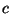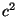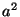# A visual proof of the Pythagorean theorem

Wolfgang Slany
http://slany.org/wolfgang/
http://www.dbai.tuwien.ac.at/proj/pf2html/

April 25, 2002

Theorem (): The area of the square built upon the hypotenuse of a right triangle is equal to the sum of the areas of the squares upon the remaining sides. Stated algebraically, for a right triangle with sides of lengths,, and, whereis the length of the hypotenuse,For example, the area of the square labeledin the following figure is equal to the sum of the areas of the squares labeledand.Proof:

## Bibliography

1
Chou pei suan ching: The Arithmetical Classic of the Gnomon and the Circular Paths of Heaven, a Chinese astronomical and mathematical treatise. Exact date of origin unknown http://www.google.com/search?q=chou+pei+suan+ching.

A visual proof of the Pythagorean theorem

This document was generated using the LaTeX2HTML translator Version 2K.1beta (1.56)

Copyright © 1993, 1994, 1995, 1996, Nikos Drakos, Computer Based Learning Unit, University of Leeds.
Copyright © 1997, 1998, 1999, Ross Moore, Mathematics Department, Macquarie University, Sydney.

The command line arguments were:
latex2html pythagoras

The translation was initiated by Glowacki Martin / TU Wien Studenten Account Kopie on 2002-10-01

This HTML file was generated using the pf2html extension, version 0.03.
LaTeX2HTML + pf.sty (hypertext proofs).

Project homepage at http://www.dbai.tuwien.ac.at/proj/pf2html

Glowacki Martin / TU Wien Studenten Account Kopie 2002-10-01